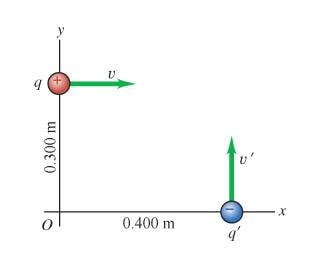# Magnetic Force and Two Moving Charges

• Soaring Crane

## Homework Statement

A pair of point charges, q = 7.60 microC and q' = -4.50 microC, are moving in a reference frame, as shown in the figure, with speeds 9.00×10^4 m/s and v' = 6.40×10^4 m/s.a. When the point charges are at the locations shown in the figure, what magnetic force does q' exert on q?

b. What is its direction?

## Homework Equations

I came across a formula for the magnetic force F_a on b:

F_ab = q_b*v_b[(mu_0/(4*pi)]*[(q_a*v_a*u_ab)/r^2]

where u_ab = unit vector directed from q_a to q_b

Shortened F_ab = q_b*v_b*B_a??

## The Attempt at a Solution

I really don’t know if I used the formula properly, so:

Magnitude B_q’ = [mu_0/(4*pi)]*[(q_a*v_b*u_ab)/r^2 = [mu_0/(4*pi)]*[(q_a*v_b*r_ab)/r^3

= (1.0*10^-7)*(32000 m^2/s)*(4.50*10^-6 C)/[0.500m]^3
= 819.2 T ??

Magnitude F_q’ on q = q*v_q*B_q’ = ((7.60*10^-6 C)*(9.00*10^4 m/s)*819.2 T) = 560.33 N ?

Will the force’s direction be in the positive(+) y-direction?

Thanks.

Can anyone please inform me if the method/direction is correct?